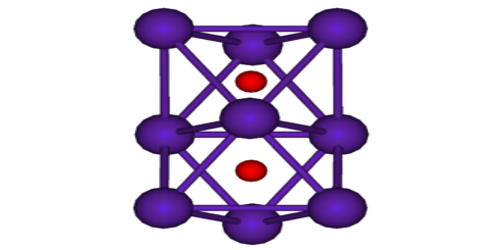Chemistry

# SuboxidesSuboxides are the oxide of an element that contains the smallest proportion of oxygen. They are a class of oxides wherein the electropositive element is in excess relative to the “normal” oxides. Sources of suboxides, providing several advantages over metal sources for the molecular beam epitaxy (MBE) of oxides, are conventionally realized by decomposing the corresponding oxide charge at extreme temperatures. When the electropositive element is a metal, the compounds are sometimes referred to as “metal-rich”. Thus the normal oxide of cesium is Cs2O, which is described as a Cs+ salt of O2−. A suboxide of cesium is Cs11O3, where the charge on Cs is clearly less than 1+, but the oxide is still described as O2−.

Suboxide is an oxide containing a relatively small amount of oxygen.  The high decomposition temperatures of the pure oxide charge were found to produce a high parasitic oxygen background. Suboxides typically feature extensive bonding between the electropositive element, often leading to clusters. In contrast, the mixed charges reacted at significantly lower temperatures, providing high suboxide fluxes without additional parasitic oxygen. It is an oxide containing a relatively small amount of oxygen, and less than the normal proportion; as, potassium suboxide, K4O. Another example – Three oxides of carbon are known, namely, carbon suboxide, C,02, carbon monoxide, CO, and carbon dioxide, C02. Carbon suboxide, C302, is formed by the action of phosphorus pentoxide on ethyl malonate.

For example, Carbon suboxide, C3O2, is a foul-smelling lacrimatory (tear-stimulating) gas produced by the dehydration of malonic acid, CH2(COOH)2, with P4O10 in a vacuum at 140 to 150 °C (284 to 302 °F). Carbon suboxide O=C=C=C=O has two infrared active stretching bands at 2258 and 1573 cm−1 and two Raman active stretching bands at 2200 and 830 cm−1. Gaseous carbon suboxide has an evil smell and while stable at –78 °C it polymerizes at 25 °C. Photolysis of C3O2 yields the unstable C2O.

Examples of suboxides include

• Carbon suboxide, C3O2;
• Boron suboxide, B6O;
• Rubidium suboxide, Rb9O2;
• Silicon suboxide, SiOx (x < 2);
• Titanium suboxide, TiOx;
• Titanium monoxide, TiO;
• Tungsten suboxide, WOx.